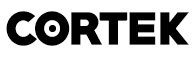# LabVIEW

cancel
Showing results for
Search instead for
Did you mean:

## The sizes of the input matrices are incompatible

Dear all,

I am having trouble solving a little part of matlab script in labview. I am multiplying two arrays ( a 2D with 1D) inside mathscript but it generates an error of input matrices incompatibilty. The sizes of the input matrices are incompatible.  Verify that the matrices have the same size or that one is a scalar.

I have tried executing same code in MATLAB and it generates no error; gives me the correct values. The code is
projected_test_image = eigenfaces'.*normalized_test_image;

Why is my labview not solving this while matlab does? Help neededRegardsMessage 1 of 14
(1,144 Views)

## Re: The sizes of the input matrices are incompatible

Hi,

attaching VI could help. Do you have the right dimension of matrix and vector?

Simyfren

Message 2 of 14
(1,133 Views)

## Re: The sizes of the input matrices are incompatible

Hello NapDynamite,

Likely what is happening is that your 1D array is of the wrong orientation. LabVIEW does not differentiate between a row vector and a column vector. Thus, MathScript must make a decision when it inputs a 1D array. It happens to choose a row vector. But even if it chose a column vector, it's wrong half the time. Thus, in your code, you are multiplying a 2D array with a row vector. This isn't allowed. Try transforming your normalized_test_image vector in your MathScript node.

See the second-to-last paragraph for reference: http://zone.ni.com/reference/en-XX/help/371361J-01/lvconcepts/scriptnodes/

Grant M.
Senior Software Engineer | LabVIEW Tablets | National Instruments

Message 3 of 14
(1,122 Views)

## Re: The sizes of the input matrices are incompatible

Dear GrantM,
I tried doing same thing by labview nodes ( transpose array and then cross-product). Now comes another situation which I couldn't just figure out. I have another matlab node as follows. I have converted each array into matrix, fed it to script inputs and it's running but not giving correct values. Before this node, I have cross checked every loop with matlab data and they are correct. But at this point the value is not correct ( Euc_dist_min ).
Do I need to change something in the script? Can you please point out?Regards.Message 4 of 14
(1,113 Views)

## Re: The sizes of the input matrices are incompatible

OR
How can I perform same operation using LabVIEW functions ( for loop, vector norm function but don't havt quite an idea ). I need to match the result from original .m script running in matlab for verification.

Regards.Message 5 of 14
(1,109 Views)

## Re: The sizes of the input matrices are incompatible

Hello NapDynamite,

Converting the 1D array into a 2D array before giving it to the MathScript node is another workaround. However, it may be simpler to avoid the 2D array and just transpose the 1D array on the first line:
normalized_test_image = normalized_test_image.';

You want to use the array transpose operator (.') to avoid calculating the Hermitian transpose ('). Although, if your values are all real, the two are identical.

As for performing the same operations in LabVIEW, the help for the norm function gives some implementation details. The default case computes the Euclidean (or l2) norm. For a vector [a b c], it is equivalently calculated by
vector_norm = sqrt(a^2 + b^2 + c^2);

Since you are squaring the result of your norm calculation, note that you no longer have the Euclidean distance.

Grant M.
Senior Software Engineer | LabVIEW Tablets | National Instruments

Message 6 of 14
(1,095 Views)

## Re: The sizes of the input matrices are incompatible

Can you guys help me with a similar problem?

I'm working with a discrete PID and I noticed that the error is in the vector xk1 so I tried to transpose it but it doesn't work either. The error suggests an incompatibility of dimensions. I read somewhere else that labview reads and displays arrays as column vectors in 2-D array real (which is the type of data that belongs xk output), while in mathscript the arrays are read as matlab code. As I said, even transposing the vectors I can't get to multiply A*xk1.P.d. Error:

Error 1050 occurred at LabVIEW: (Hex 0x441) Error occurred while executing script. Error message from server: ??? Error using *
Incorrect dimensions for matrix multiplication. Check that the number of columns in the first matrix matches the number of rows in the second matrix. To perform elementwise multiplication, use '.*'.

Message 7 of 14
(92 Views)

## Re: The sizes of the input matrices are incompatible

Hi Jose,

there is a full palette of Matrix operations in the Math functions.

I don't know why you need a MATLAB node for such basic math…

Best regards,
GerdW
CLAD expired, using 2009SP1 + LV2011SP1 + LV2017 (+LV2018 sometimes) on Win7+cRIO
Kudos are welcomeMessage 8 of 14
(74 Views)

## Re: The sizes of the input matrices are incompatible

Because that's  the only way to get xk and generate the output "y" for the system when working in space-state. Please, help me out with a solution..

Message 9 of 14
(65 Views)

## Re: The sizes of the input matrices are incompatible

Hi Jose,

can you downconvert your VI to LV2017 and attach it again?

Best regards,
GerdW
CLAD expired, using 2009SP1 + LV2011SP1 + LV2017 (+LV2018 sometimes) on Win7+cRIO
Kudos are welcomeMessage 10 of 14
(58 Views)